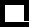The Krib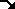Chemistry [E-mail]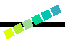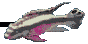# Water Changes

## 25% Water Changes & Ultimate Concentration of Junk/Nutrients

### by rjw-at-aluxs.micro.lucent.com (Ronald Wozniak) Date: Mon, 18 Nov 96

```I hear over and over again this "aquarium rule of thumb" in directions
for adding X mg/liter of PPDD, magnesium, or whatever to add to your
aquarium that "you can't overdose as long as you do 25% water changes every
two weeks". (PPDD == Poor Persons Dupla Drops)

At first, it seemed to me, by adding some material every day/week and then
only taking out 25% of it leaving 3/4 of it (with a 25% water change) every
two weeks, that one would build up stuff until one exceeds some limit and
things in your aquarium would start dying. [Wow - say that last sentence ten
times without breathing.]

Anyway, for some people this "aquarium rule of thumb" may seem obvious, but
for me it wasn't.  So after a little digging around, I can now try to explain
why this rule seems to be a "fact" and not a "myth".

First, let's say we add 50mg/day of material (NaCl) to a 700 liters aquarium
for two weeks, then do a 25% water change.  After we continue doing this for
a number of weeks, what would be the eventual concentration of this material
(NaCl) in the water? Of course, we need to assume that the living
inhabitants or nonliving whatevers are _not_ consuming/storing this material
so that it can removed by a water change.  On the other hand, if we know that
we add 60mg of nitrogen a day and somehow we figure out that bacteria are
consuming 10mg of this nitrogen each day, then we use the net 50mg/day for
our calculations.

Table 1.  Let's See What's Happening So Far...

Before Water Change             After Water Change

Time     Total Amount - Concentration    Total Amount - Concentration
- ----     ------------   -------------    ------------   -------------

2nd Week  700mg            1mg/L            525mg         0.75 mg/L
[50mg*14days] [700mg/700L]      [700*3/4]       [525/700]

4th Week  1225mg         1.75mg/L           919mg          1.31mg/L
[525 + 700]  [1225mg/700L]      [1225*3/4]      [919/700]

6th Week  1619mg         2.31mg/L          1214mg          1.73mg/L
[919 + 700]  [1619mg/700L]      [1619*3/4]      [1214/700]

8th Week  1914mg         2.73mg/L          1435mg          2.05mg/L
[1214 + 700]  [1914mg/700L]      [1914*3/4]      [1435/700]

At this point, it's not clear what the final amounts are going to be.
One may observe that in the Before Water Change that the _difference_ in
Concentration from one water change to the previous water change seems to
be going down: (Likewise for the After Water Change Concentration.)

4th Week - 2nd Week => 1.75 mg/L - 1mg/L    = 0.75 mg/L
6th Week - 4nd Week => 2.31 mg/L - 1.75mg/L = 0.56 mg/L
8th Week - 6nd Week => 2.73 mg/L - 2.31mg/L = 0.42 mg/L

However, a pattern (series) is starting to form:

The total amount this week          total amount last week + 700
before water change         =        after water change

and

The total amount this week           total amount this week * 3/4
after water change          =       before water change

If we let X be the net amount of material we add before every water change,
and (1-r)*100  be the percent water change over this same time period.
(e.g. For 25% water change r=3/4, or for 20% water change r=4/5)

We have:

Before Water Change           After Water Change

Time           Total Amount                  Total Amount
- ----           ------------                  ------------

1 period      X      units                X(r)     units

2 period      X(1 + r)  units             X(r + r*r) units
[X*r + X]                   [(X*r + X)*r]

3 period      X(1 + r + r*r)              X(r + r^2 + r^3)
4 period      X(1 + r + r^2 + r^3)        X(r + r^2 + r^3 + r^4)
5 period      X(1 + r + r^2 + r^3 + r^4)  X(r + r^2 + r^3 + r^4 + r^5)

etc.....

where:

r^2 = r*r
r^3 = r*r*r
r^4 = r*r*r*r
etc
r^n = r*r*r* etc... *r } n terms of r

Some people may recognize (1 + r + r^2 + *** + r^(n-1) + r^n)

where 0 < r < 1,

as the well known, at least to Math majors, Geometric Series.

- ----------------------------------------------------------------

Geometric Series:

For n time periods, (1 + r + r^2 + *** + r^(n-1)) = (1 - r^n)
---------
1 - r

which, after a long time, n get real big, (i.e., n approaches infinity)

(1 - r^n)                   1
----------     becomes    -----   where 0 < r < 1
1 - r                    1 - r

- ----------------------------------------------------------------
So for the nth water change:

Before Water Change           After Water Change

Time             Total Amount                  Total Amount
- ----             ------------                  ------------

nth period          (1 - r^n)                     (1 - r^n))
X *  ---------                X *  ---------  * r
1 - r                         1 - r

When n gets big,  the r^n terms get very small, so after a long time

Before Water Change           After Water Change

Time             Total Amount                  Total Amount
- ----             ------------                  ------------

long time                 1                           r
X *  -----                   X * -----
1 - r                       1 - r

>From this, we can construct the following table.  With X net units of material
of material in the aquarium can be found.

Table 2. The Ultimate (Steady State) Amount of Material In the Aquarium
by Adding X amount Before/After Each % Water Change

Before Water Change        After Water Change
Ultimate                   Ultimate
% Water Change        Total Amount               Total Amount
- --------------     -------------------        ------------------

10% (r=9/10)             10 * X                     9 * X

20% (r=4/5)               5 * X                     4 * X

25% (r=3/4)               4 * X                     3 * X

33% (r=2/3)               3 * X                     2 * X

50% (r=1/2)               2 * X                       X

66% (r=1/3)               3/2 * X                  1/2 * X

75% (r=1/4)               4/3 * X                  1/3 * X

Smoke and mirrors one may say!  Basically what's happening is that material
builds up in the aquarium until the amount we put in before each water change
is equal to the amount one takes out during the water change.

So we know what the ultimate (steady state) amount of material will be in
the aquarium after "a long enough" period of time (water changes). But, one may
ask, how long is "a long enough" period of time?

Let's compute the number of water changes it takes so that the total amount of
material in the aquarium reaches within 20%, 10%, or 5% of the steady state
value.

All we got do is, for a given r,  solve for n when:

(1 - r^n)
---------  =  0.8 or 0.9 or 0.95
1 - r

Table 3. For a given Percent water Change, the Number of Water changes
to 80%, 90%, or 95% Of the Steady State Amount

Number of Water   Number of Water  Number of Water
Changes to 80%    Changes to 90%   Changes to 95%
% water change       Total Amount      Total Amount    Total Amount
- --------------     ---------------   ---------------  ---------------

10% (r=9/10)             16                22               29

20% (r=4/5)               8                11               14

25% (r=3/4)               6                 9               11

33% (r=2/3)               4                 6                8

50% (r=1/2)               3                 4                5

66% (r=1/3)               2                 3                3

75% (r=1/4)               2                 2                3

It's interesting to note that for a 10% water change, the steady state
variation
in the amount of material before and after water change is only 10%, but it
takes
about a year (for water changes every two weeks) to get there. For a 50% water
change,
it only takes about two months (4 water changes) to get close to the steady
state,
but one gets a 50% variation in the amount of material that being added between
every
water change.  The 25% water change seems to be a pretty good compromise
between
time to steady state and concentration variation between water changes.

Of course, if one knew how much to add, one could just add the amount needed to
reach
the desired steady state, and then add just the right amount at water change to
keep the
total concentration constant. (i.e. It's not too difficult to measure the KH of
the water
before the water change, and using George Booth's recipe of "one teaspoon
of sodium bicarbonate (NaHCO3) per 50 liters will increase KH by 4 degrees and
will not
increase general hardness," one could adjust the water to the desired level.)
On the
other hand, it's difficult to determine which micronutrient may be overdosing
the plants
before it's too late. So, at least with frequent water changes, and knowing
what you're
adding, one can at least keep the micronutrients below a certain amount.

Sorry for the long post, but I hope some people find this information useful.

Ron Wozniak  Allentown PA, USA
rjwozniak-at-lucent.com

```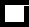Up to Chemistry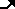The Krib This page was last updated 29 October 1998